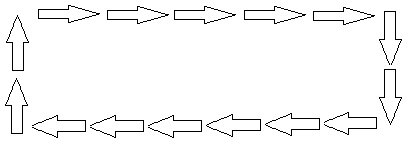KCC3
 Name:    KCC3

 CompletionComplete each statement. 1. Count how many dots there are.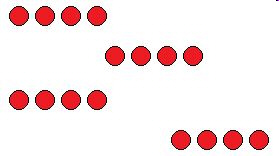2. Count how many animals there are.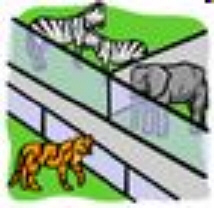3. Count how many peas are in the pod?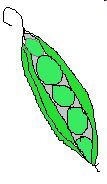4. Count how many flowers there are.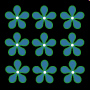5. Count how many black dots are there in the square?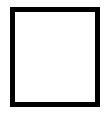6. Count how many red dots are there?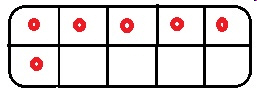7. How many white dots are there on the dominos?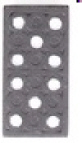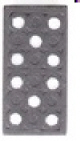8. How many arrows are there?